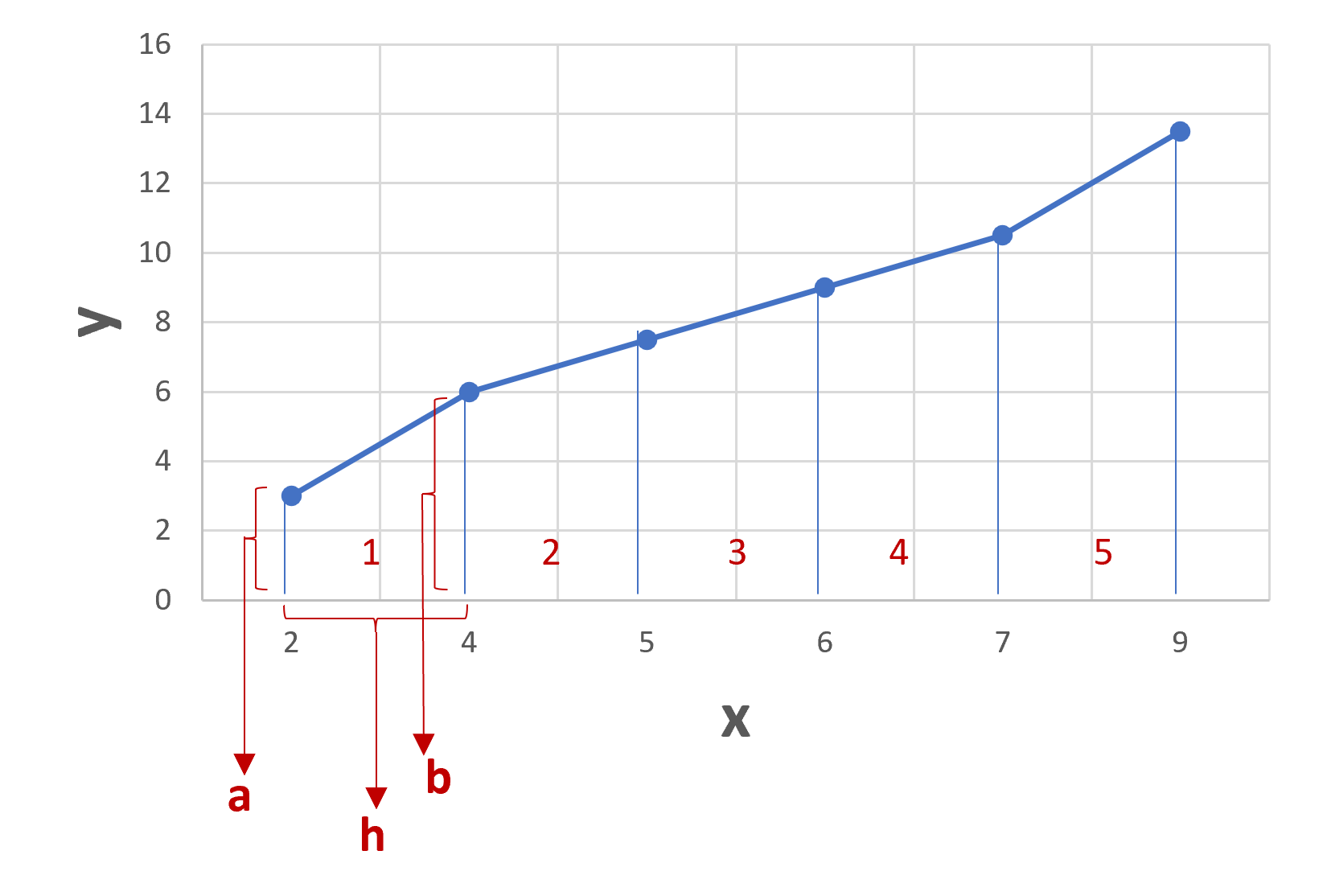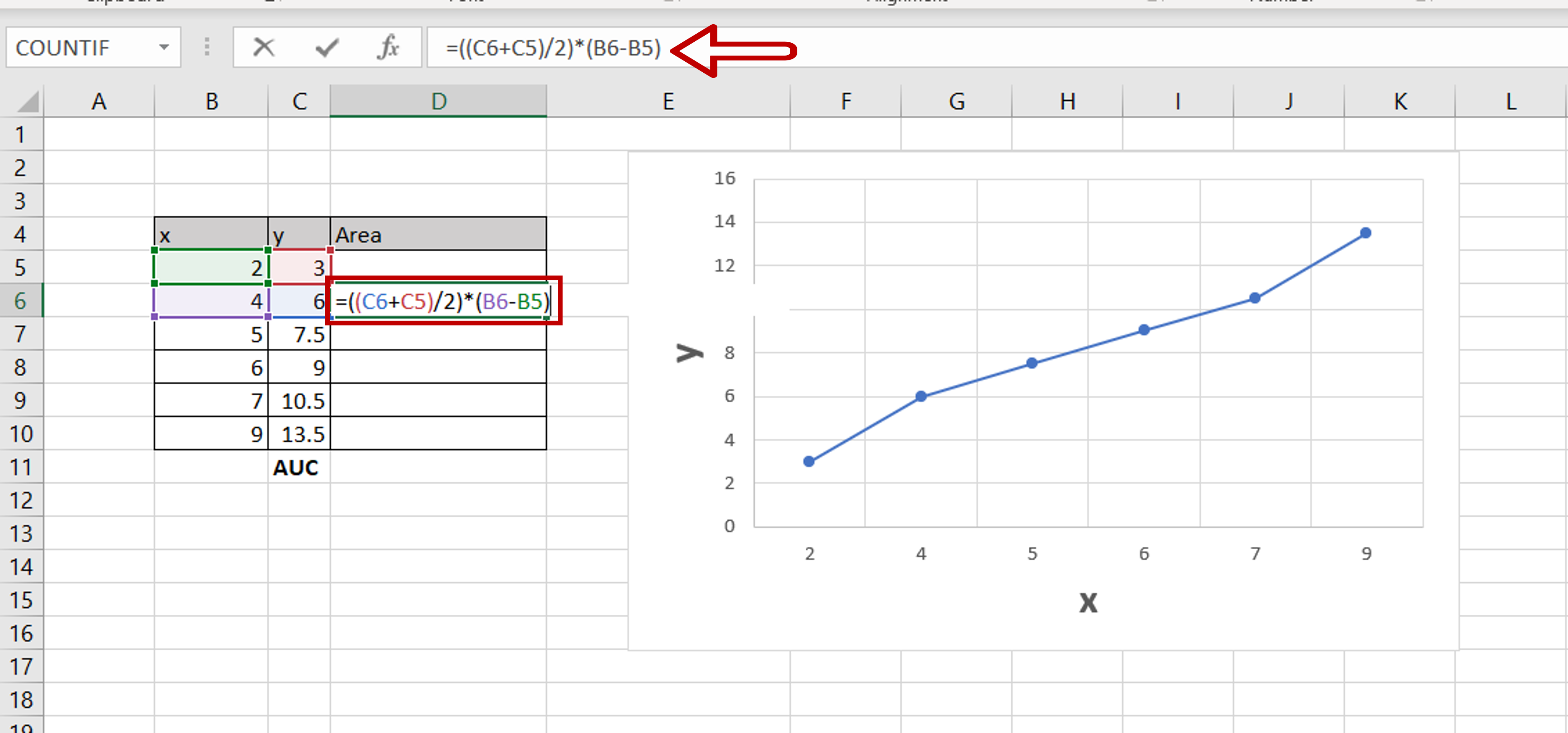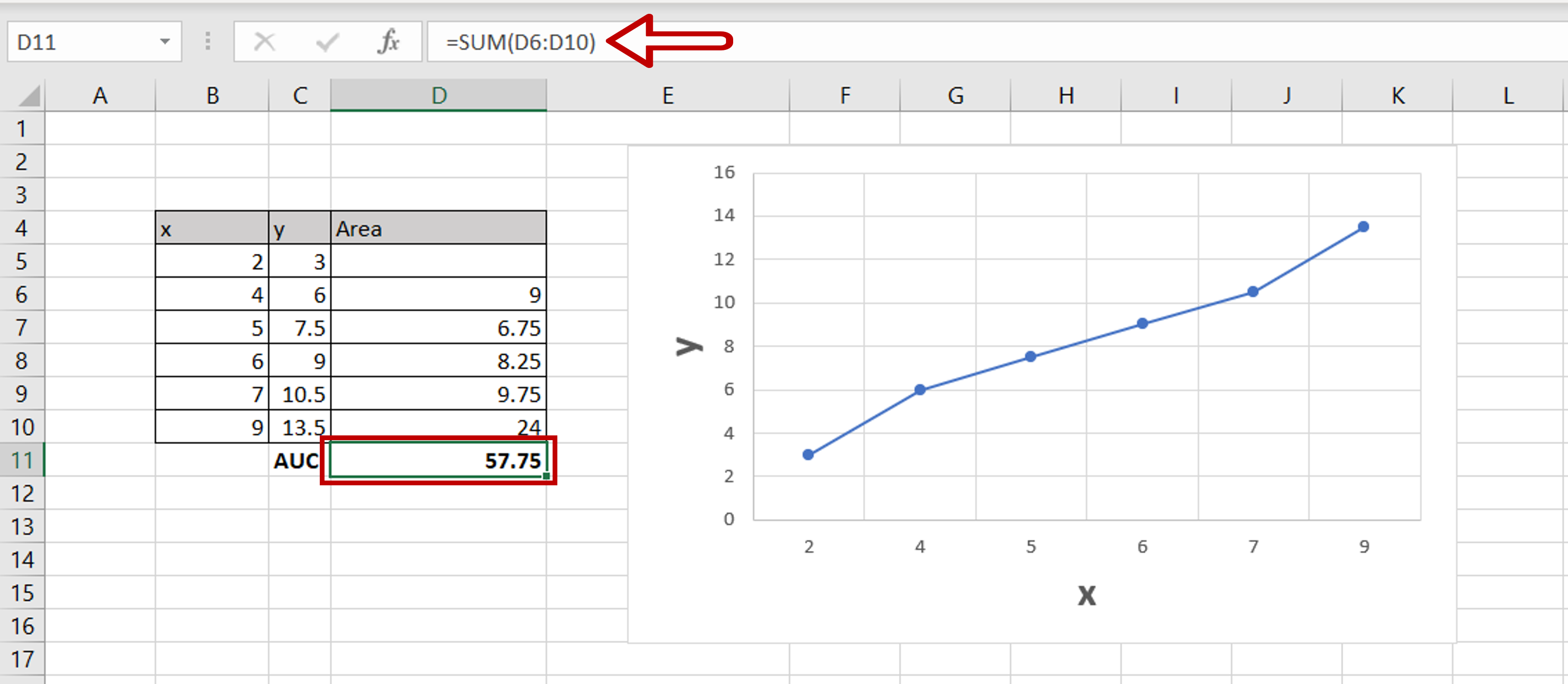# How to calculate the area under curve in Excel

You can watch a video tutorial here.Area Under Curve or AUC is widely used in Data Science. It is the area between a plotted line and the axes. Excel does not have a function to compute this directly, but you can use formulas in Excel to derive it. The approach is to look at the area under the curve as a set of trapezoids, bound by the line on one side and the axis on the other. This is shown in the image above

The area under the curve is divided into 5 trapezoids. We will calculate the area of each trapezoid and add them all up. This will give us the AUC. The formula we will use for the area is:
Area = ((a+b)/2)*h
a = one of the parallel sides
b = the other parallel side
h = the height

Using trapezoid 1 as an example,
a = the first value of y
b = the second value of y
h = the second value of x – the first value of x.

### Step 1 – Create the formula– Select the second cell in the column adjacent to the data
– Type the formula using cell references:
=  (first value of y + second value of y)/2*(second value of x – first value of x)
– Press Enter

### Step 2 – Copy the formula– Using the fill handle from the first cell, drag the formula to the remaining cells
OR
a) Select the cell with the formula and press Ctrl+C or choose Copy from the context menu (right-click)
b) Select the rest of the cells in the column and press Ctrl+V or choose Paste from the context menu (right-click)

### Step 3 – Sum up the areas– Select the cell at the end of the column with the areas
– Type the formula using cell references:
=SUM(range of the area column)
– Press Enter
– The area under the curve is computed